# Writing and Solving one Step Equations

•WatchVariables and Expressions
•WatchOrder of Operations
•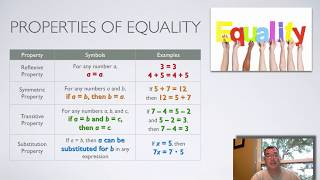WatchProperties of Numbers
•WatchDistributive Property
•WatchEquations
•WatchWriting Equations
•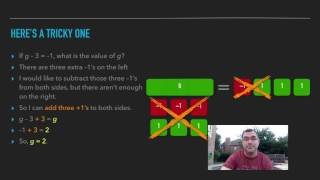WatchSolving One-Step Equations
•WatchSolving Two-Step Equations
•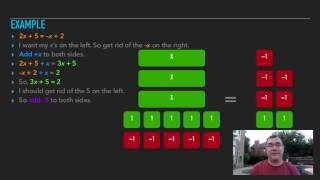WatchSolving Equations with the Variable on Both Sides
•WatchLiteral Equations and Dimensional Analysis
•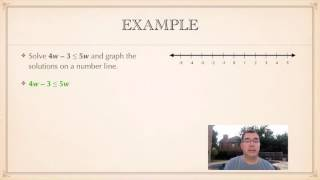WatchSolving Inequalities by Addition and Subtraction
•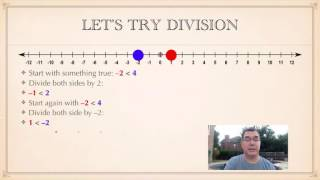WatchSolving Inequalities by Multiplication and Division
•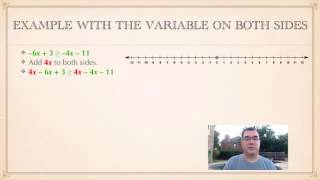WatchSolving Multi-Step Inequalities

Writing and Solving 1-Step Integer Equations. A one-step equation is as straightforward as it sounds. You will only need to perform one step in order to solve the equation. For example, addition “undoes” subtraction and subtraction “undoes” addition.